Next: Paired -tests Up: Examples Previous: Numerical simulation

## Repeated Measures

In the case of repeated measures, where some or all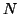subjects were scanned more than once, we might want to account for a possible difference in the between-subject variance and the between-session variance of the same subject. Thus, we simply extend the previous model to write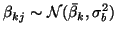and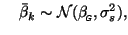where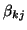denotes the first-level parameter for subject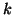obtained at session,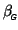is the population mean,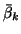is the subject-specific mean,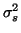is the between-subject variance and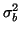is the between-session variance. Note that this can be extended to the case of repeated measures under different conditions by introducing more variance terms. The existence of these additional variance terms will be reflected in the covariance matrix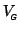simply by allowing for off-diagonal elements. As an example, consider the case of 3 subjects each measured twice, then with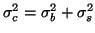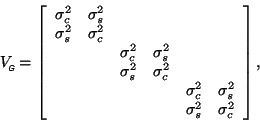where, for simplicity, we order the subjects in pairs appropriately. Hence, we can simply adjust any covariance structureat the group level to take account of the existence of repeated measurements. Alternatively, note that because our previous results extend to multi-level GLMs, we can simply combine the different sessions per subject on a second level and calculate group parameters of interest in a third level.Next: Paired -tests Up: Examples Previous: Numerical simulation
Christian Beckmann 2003-07-16